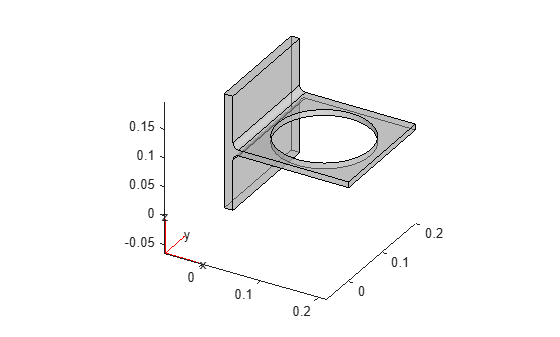structuralProperties

Assign structural properties of material for structural model

Description

structuralProperties(structuralmodel,'YoungsModulus',YMval,'PoissonsRatio',PRval) assigns the Young's modulus and Poisson's ratio for the entire geometry. Use this syntax if your model is static and does not account for gravitational and thermal effects.

Tip

A structural model supports only homogeneous isotropic materials. Therefore, all material properties must be numeric scalars.

example

structuralProperties(___,'MassDensity',MDval) assigns the mass density of the material for the entire geometry, and can include any of the arguments used in the previous syntax. Specify the mass density of the material if your model is transient or modal, or if it accounts for gravitational effects.

example

structuralProperties(___,'CTE',CTEval) assigns the coefficient of thermal expansion for a thermal stress analysis. Use this syntax if your model is static and accounts for thermal effects.

example

structuralProperties(___,RegionType,RegionID) assigns material properties for the specified geometry region.

mtl = structuralProperties(___) returns the material properties object.

Examples

collapse all

Create a structural model.

structuralModel = createpde('structural','static-solid');

Import and plot the geometry.

importGeometry(structuralModel,'BracketWithHole.stl');
pdegplot(structuralModel,'FaceAlpha',0.5)Specify the Young's modulus, Poisson's ratio, and mass density.

structuralProperties(structuralModel,'YoungsModulus',200e9, ...
'PoissonsRatio',0.3, ...
'MassDensity',7800)
ans =
StructuralMaterialAssignment with properties:

RegionType: 'Cell'
RegionID: 1
YoungsModulus: 2.0000e+11
PoissonsRatio: 0.3000
MassDensity: 7800
CTE: []

Create a structural model for modal analysis.

structuralModel = createpde('structural','modal-solid');

Create and plot the geometry.

gm = multicuboid(0.5,0.1,0.1);
structuralModel.Geometry = gm;
pdegplot(structuralModel,'FaceAlpha',0.5)Specify the Young's modulus, Poisson's ratio, and mass density.

structuralProperties(structuralModel,'YoungsModulus',210E3, ...
'PoissonsRatio',0.3, ...
'MassDensity',2.7E-6)
ans =
StructuralMaterialAssignment with properties:

RegionType: 'Cell'
RegionID: 1
YoungsModulus: 210000
PoissonsRatio: 0.3000
MassDensity: 2.7000e-06
CTE: []

Specify the coefficients of thermal expansion for a bimetallic cantilever beam. The bottom layer is steel. The top layer is copper.

Create a static structural model.

structuralmodel = createpde('structural','static-solid');

Create and plot the geometry.

gm = multicuboid(0.5,0.04,[0.03,0.03],'Zoffset',[0,0.03]);
structuralmodel.Geometry = gm;
pdegplot(structuralmodel,'CellLabels','on')Specify the Young's modulus, Poisson's ratio, and coefficient of thermal expansion for the bottom cell C1.

structuralProperties(structuralmodel,'Cell',1', ...
'YoungsModulus',210e9, ...
'PoissonsRatio',0.28, ...
'CTE',1.3e-5)
ans =
StructuralMaterialAssignment with properties:

RegionType: 'Cell'
RegionID: 1
YoungsModulus: 2.1000e+11
PoissonsRatio: 0.2800
MassDensity: []
CTE: 1.3000e-05

Specify the Young's modulus, Poisson's ratio, and coefficient of thermal expansion for the top cell C2.

structuralProperties(structuralmodel,'Cell',2', ...
'YoungsModulus',110e9, ...
'PoissonsRatio',0.37, ...
'CTE',2.4e-5)
ans =
StructuralMaterialAssignment with properties:

RegionType: 'Cell'
RegionID: 2
YoungsModulus: 1.1000e+11
PoissonsRatio: 0.3700
MassDensity: []
CTE: 2.4000e-05

Create a structural model.

structuralModel = createpde('structural','static-solid');

Create nested cylinders to model a bimetallic cable.

gm = multicylinder([0.01,0.015],0.05);

Assign the geometry to the structural model and plot the geometry.

structuralModel.Geometry = gm;
pdegplot(structuralModel,'CellLabels','on','FaceAlpha',0.4)Specify the Young's modulus and Poisson's ratio for each metal.

structuralProperties(structuralModel,'Cell',1,'YoungsModulus',110E9, ...
'PoissonsRatio',0.28)
ans =
StructuralMaterialAssignment with properties:

RegionType: 'Cell'
RegionID: 1
YoungsModulus: 1.1000e+11
PoissonsRatio: 0.2800
MassDensity: []
CTE: []

structuralProperties(structuralModel,'Cell',2,'YoungsModulus',210E9, ...
'PoissonsRatio',0.3)
ans =
StructuralMaterialAssignment with properties:

RegionType: 'Cell'
RegionID: 2
YoungsModulus: 2.1000e+11
PoissonsRatio: 0.3000
MassDensity: []
CTE: []

Input Arguments

collapse all

Structural model, specified as a StructuralModel object. The model contains the geometry, mesh, structural properties of the material, body loads, boundary loads, and boundary conditions.

Example: structuralmodel = createpde('structural','transient-solid')

Young's modulus of the material, specified as a positive number.

Example: structuralProperties(structuralmodel,'YoungsModulus',210e3,'PoissonsRatio',0.3)

Data Types: double

Poisson's ratio of the material, specified as a number greater than 0 and less than 0.5.

Example: structuralProperties(structuralmodel,'YoungsModulus',210e3,'PoissonsRatio',0.3)

Data Types: double

Mass density of the material, specified as a positive number. This argument is required for transient and modal models. MDval is also required when modeling gravitational effects.

Example: structuralProperties(structuralmodel,'YoungsModulus',210e3,'PoissonsRatio',0.3,'MassDensity',11.7e-6)

Data Types: double

Coefficient of thermal expansion, specified as a real number. This argument is required for thermal stress analysis. Thermal stress analysis requires the structural model to be static.

Example: structuralProperties(structuralmodel,'YoungsModulus',210e3,'PoissonsRatio',0.3,'MassDensity',2.7e-6,'CTE',11.7e-6)

Data Types: double

Geometric region type, specified as 'Face' for a 2-D model or 'Cell' for a 3-D model.

Example: structuralProperties(structuralmodel,'Cell',1,'YoungsModulus',110E9,'PoissonsRatio',0.3)

Data Types: char | string

Geometric region ID, specified as a vector of positive integers. Find the region IDs using pdegplot with the 'FaceLabels' (3-D) or 'EdgeLabels' (2-D) value set to 'on'.

Example: structuralProperties(structuralmodel,'Cell',1:3,'YoungsModulus',110E9,'PoissonsRatio',0.3)

Data Types: double

Output Arguments

collapse all

Handle to material properties, returned as a StructuralMaterialAssignment object. See StructuralMaterialAssignment Properties.

mtl associates the material properties with the geometric region.

Introduced in R2017b

Partial Differential Equation Toolbox Documentation